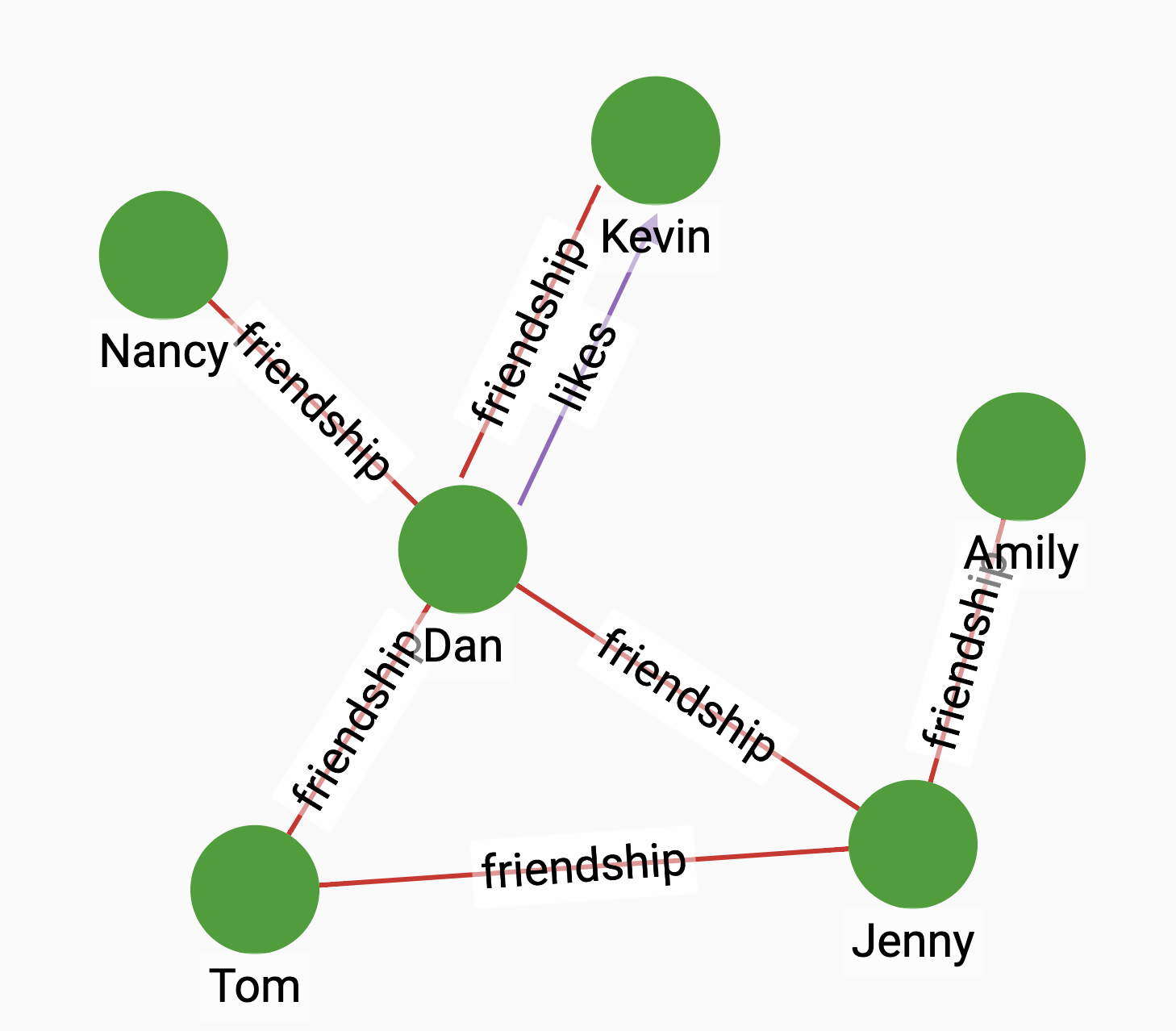# Total Neighbors

The algorithm calculates the total number of neighbors of two vertices.

## Specifications

``````CREATE QUERY tg_total_neighbors(VERTEX a, VERTEX b,
SET<STRING> e_type)``````

### Time complexity

The algorithm has a time complexity of O(D1 + D2), where D1 and D2 are the degrees of the two vertices.

## Parameters

Name Description Data type

`a`

A vertex.

`VERTEX`

`b`

A vertex.

`VERTEX`

`e_type`

Edge types to traverse.

`SET<STRING>`

## Return value

The total number of neighbors of two vertices.

## Example

Suppose we have the following graph.Dan and Jenny together have 6 neighbors in total. Running the algorithm between Dan and Jenny would give us a result of 6. Note that since Jenny and Dan are neighbors themselves, the union of their neighbors includes both Jenny and Dan:

• Query

• Result

``RUN QUERY tg_total_neighbors (("Jenny", "person"), ("Dan", "person"), ["friendship"])``
``````{
"error": false,
"message": "",
"version": {
"schema": 2,
"edition": "enterprise",
"api": "v2"
},
"results": [{"closeness": 6}]
}``````